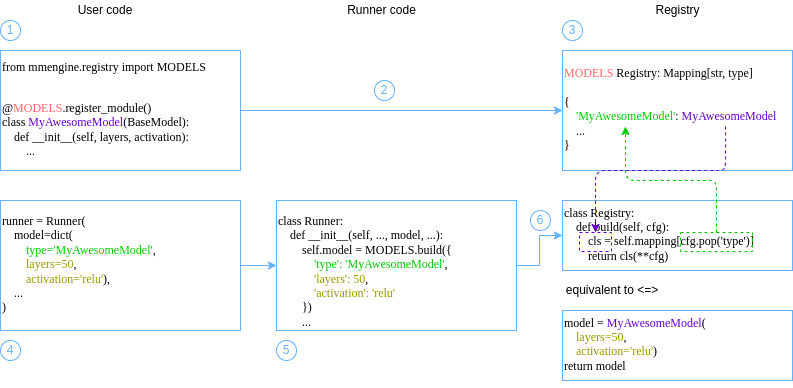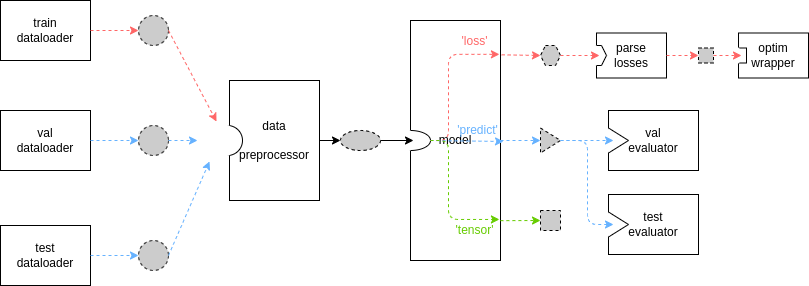Shortcuts

# 执行器（Runner）¶

• 掌握执行器的常见参数与使用方式

• 了解执行器的最佳实践——配置文件的写法

• 了解执行器基本数据流与简要执行逻辑

• 亲身感受使用执行器的优越性（也许）

## 执行器示例¶

• 参考 API 文档，逐项确认和配置参数

• 在已有配置（如 15 分钟上手MMDet 等下游算法库）的基础上，进行定制化修改

### 面向初学者的示例代码¶

import torch
import torch.nn as nn
import torch.nn.functional as F
from torch.utils.data import Dataset

from mmengine.model import BaseModel
from mmengine.evaluator import BaseMetric
from mmengine.registry import MODELS, DATASETS, METRICS

@MODELS.register_module()
class MyAwesomeModel(BaseModel):
def __init__(self, layers=4, activation='relu') -> None:
super().__init__()
if activation == 'relu':
act_type = nn.ReLU
elif activation == 'silu':
act_type = nn.SiLU
elif activation == 'none':
act_type = nn.Identity
else:
raise NotImplementedError
sequence = [nn.Linear(2, 64), act_type()]
for _ in range(layers-1):
sequence.extend([nn.Linear(64, 64), act_type()])
self.mlp = nn.Sequential(*sequence)
self.classifier = nn.Linear(64, 2)

def forward(self, data, labels, mode):
x = self.mlp(data)
x = self.classifier(x)
if mode == 'tensor':
return x
elif mode == 'predict':
return F.softmax(x, dim=1), labels
elif mode == 'loss':
return {'loss': F.cross_entropy(x, labels)}

@DATASETS.register_module()
class MyDataset(Dataset):
def __init__(self, is_train, size):
self.is_train = is_train
if self.is_train:
torch.manual_seed(0)
self.labels = torch.randint(0, 2, (size,))
else:
torch.manual_seed(3407)
self.labels = torch.randint(0, 2, (size,))
r = 3 * (self.labels+1) + torch.randn(self.labels.shape)
theta = torch.rand(self.labels.shape) * 2 * torch.pi
self.data = torch.vstack([r*torch.cos(theta), r*torch.sin(theta)]).T

def __getitem__(self, index):
return self.data[index], self.labels[index]

def __len__(self):
return len(self.data)

@METRICS.register_module()
class Accuracy(BaseMetric):
def __init__(self):
super().__init__()

def process(self, data_batch, data_samples):
score, gt = data_samples
self.results.append({
'batch_size': len(gt),
'correct': (score.argmax(dim=1) == gt).sum().cpu(),
})

def compute_metrics(self, results):
total_correct = sum(r['correct'] for r in results)
total_size = sum(r['batch_size'] for r in results)
return dict(accuracy=100*total_correct/total_size)


from torch.utils.data import DataLoader, default_collate
from mmengine.runner import Runner

runner = Runner(
# 你的模型
model=MyAwesomeModel(
layers=2,
activation='relu'),
# 模型检查点、日志等都将存储在工作路径中
work_dir='exp/my_awesome_model',

# 训练所用数据
dataset=MyDataset(
is_train=True,
size=10000),
shuffle=True,
collate_fn=default_collate,
batch_size=64,
pin_memory=True,
num_workers=2),
# 训练相关配置
train_cfg=dict(
by_epoch=True,   # 根据 epoch 计数而非 iteration
max_epochs=10,
val_begin=2,     # 从第 2 个 epoch 开始验证
val_interval=1), # 每隔 1 个 epoch 进行一次验证

# 优化器封装，MMEngine 中的新概念，提供更丰富的优化选择。
# 通常使用默认即可，可缺省。有特殊需求可查阅文档更换，如
# 'AmpOptimWrapper' 开启混合精度训练
optim_wrapper=dict(
optimizer=dict(
lr=0.001)),
# 参数调度器，用于在训练中调整学习率/动量等参数
param_scheduler=dict(
type='MultiStepLR',
by_epoch=True,
milestones=[4, 8],
gamma=0.1),

# 验证所用数据
dataset=MyDataset(
is_train=False,
size=1000),
shuffle=False,
collate_fn=default_collate,
batch_size=1000,
pin_memory=True,
num_workers=2),
# 验证相关配置，通常为空即可
val_cfg=dict(),
# 验证指标与验证器封装，可自由实现与配置
val_evaluator=dict(type=Accuracy),

# 以下为其他进阶配置，无特殊需要时尽量缺省
# 钩子属于进阶用法，如无特殊需要，尽量缺省
default_hooks=dict(
# 最常用的默认钩子，可修改保存 checkpoint 的间隔
checkpoint=dict(type='CheckpointHook', interval=1)),

# luancher 与 env_cfg 共同构成分布式训练环境配置
launcher='none',
env_cfg=dict(
cudnn_benchmark=False,   # 是否使用 cudnn_benchmark
backend='nccl',   # 分布式通信后端
mp_cfg=dict(mp_start_method='fork')),  # 多进程设置
log_level='INFO',

# 加载权重的路径 (None 表示不加载)
# 从加载的权重文件中恢复训练
resume=False
)

# 开始训练你的模型吧
runner.train()


### 示例代码讲解¶

from mmengine.model import BaseModel
from mmengine.runner import Runner
from mmengine.registry import MODELS # 模型根注册器，你的自定义模型需要注册到这个根注册器中

@MODELS.register_module() # 用于注册的装饰器
class MyAwesomeModel(BaseModel): # 你的自定义模型
def __init__(self, layers=18, activation='silu'):
...

# 基于注册机制的例子
runner = Runner(
model=dict(
type='MyAwesomeModel',
layers=50,
activation='relu'),
...
)

# 基于手动构建的例子
model = MyAwesomeModel(layers=18, activation='relu')
runner = Runner(
model=model,
...
)OpenMMLab 下游库广泛采用了配置文件的方式。我们将在下个章节，基于上述示例稍微变换，从而展示配置文件——MMEngine 中执行器的最佳实践——的用法。

## 执行器最佳实践——配置文件¶

MMEngine 提供了一套支持 Python 语法的、功能强大的配置文件系统。你可以从之前的示例代码中近乎（我们将在下面说明）无缝地转换到配置文件。下面给出一段示例代码：

# 以下代码存放在 example_config.py 文件中
# 基本拷贝自上面的示例，并将每项结尾的逗号删去
model = dict(type='MyAwesomeModel',
layers=2,
activation='relu')
work_dir = 'exp/my_awesome_model'

dataset=dict(type='MyDataset',
is_train=True,
size=10000),
sampler=dict(
type='DefaultSampler',
shuffle=True),
collate_fn=dict(type='default_collate'),
batch_size=64,
pin_memory=True,
num_workers=2)
train_cfg = dict(
by_epoch=True,
max_epochs=10,
val_begin=2,
val_interval=1)
optim_wrapper = dict(
optimizer=dict(
lr=0.001))
param_scheduler = dict(
type='MultiStepLR',
by_epoch=True,
milestones=[4, 8],
gamma=0.1)

dataset=dict(type='MyDataset',
is_train=False,
size=1000),
sampler=dict(
type='DefaultSampler',
shuffle=False),
collate_fn=dict(type='default_collate'),
batch_size=1000,
pin_memory=True,
num_workers=2)
val_cfg = dict()
val_evaluator = dict(type='Accuracy')

default_hooks = dict(
checkpoint=dict(type='CheckpointHook', interval=1))
launcher = 'none'
env_cfg = dict(
cudnn_benchmark=False,
backend='nccl',
mp_cfg=dict(mp_start_method='fork'))
log_level = 'INFO'
resume = False


from mmengine.config import Config
from mmengine.runner import Runner
config = Config.fromfile('example_config.py')
runner = Runner.from_cfg(config)
runner.train()


## 基本数据流¶# 训练过程
data_batch = data_preprocessor(data_batch)
if isinstance(data_batch, dict):
losses = model.forward(**data_batch, mode='loss')
elif isinstance(data_batch, (list, tuple)):
losses = model.forward(*data_batch, mode='loss')
else:
raise TypeError()

# 验证过程
data_batch = data_preprocessor(data_batch)
if isinstance(data_batch, dict):
outputs = model.forward(**data_batch, mode='predict')
elif isinstance(data_batch, (list, tuple)):
outputs = model.forward(**data_batch, mode='predict')
else:
raise TypeError()
evaluator.process(data_samples=outputs, data_batch=data_batch)


• data_preprocessor 的输出需要经过解包后传递给 model

• evaluator 的 data_samples 参数接收模型的预测结果，而 data_batch 参数接收 dataloader 的原始数据

15 分钟上手对此有一定的描述，你需要在自定义模型的 forward 函数中实现 3 条数据通路，适配训练、验证等不同需求。模型文档中对此有详细解释。

## 为什么使用执行器（可选）¶

1. 你可以轻易地在已搭建流程上修改/添加所需配置，而不会搅乱整个代码。也许你起初只有单卡训练，但你随时可以添加1、2行的分布式配置，切换到多卡甚至多机训练

2. 你可以享受 MMEngine 不断引入的新特性，而不必担心后向兼容性。混合精度训练、可视化、崭新的分布式训练方式、多种设备后端……我们会在保证后向兼容性的前提下不断吸收社区的优秀建议与前沿技术，并以简洁明了的方式提供给你

3. 你可以集中关注并实现自己的惊人想法，而不必受限于其他恼人的、不相关的细节。执行器的缺省值会为你处理绝大多数的情况

## 下一步的建议¶

• 左侧的“常用功能”中包含更多常用的与新特性的示例代码可供参考

• “进阶教程”中有更多面向资深开发者的内容，可以更加灵活地配置训练流程、日志、可视化等

• 如果以上所有内容都无法实现你的新想法，那么钩子（Hook）值得一试

• 欢迎在我们的 讨论版 中发起话题求助！

© Copyright 2022, mmengine contributors. Revision c863e8b1.

Built with Sphinx using a theme provided by Read the Docs.
Versions
latest
stable
v0.8.5
v0.8.4
v0.8.3
v0.8.2
v0.8.1
v0.8.0
v0.7.4
v0.7.3
v0.7.2
v0.7.1
v0.7.0
v0.6.0
v0.5.0
v0.4.0
v0.3.0
v0.2.0